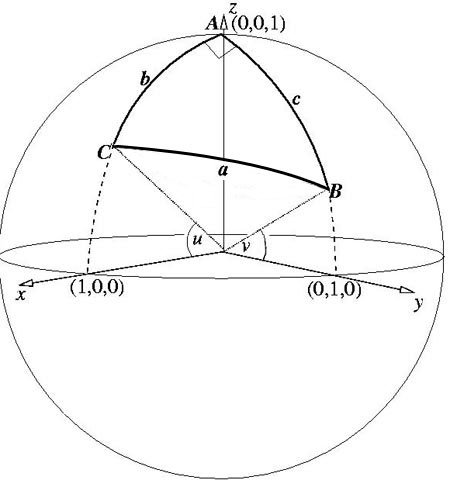#### You may also likeA quadrilateral changes shape with the edge lengths constant. Show the scalar product of the diagonals is constant. If the diagonals are perpendicular in one position are they always perpendicular?As a quadrilateral Q is deformed (keeping the edge lengths constnt) the diagonals and the angle X between them change. Prove that the area of Q is proportional to tanX.### Air Routes

Find the distance of the shortest air route at an altitude of 6000 metres between London and Cape Town given the latitudes and longitudes. A simple application of scalar products of vectors.

# Pythagoras on a Sphere

##### Age 16 to 18 Challenge Level:Many thanks Andrei from Tudor Vianu National College, Bucharest, Romania for another excellent solution. To solve the problem I have used the hint, so that all notations are from the hint. I have associated to the sphere a system of Cartesian coordinates, as shown in the sketch. Without loss of generality, I have assumed that $A$ is situated on $Oz$, and has coordinates (0, 0, 1). As $A$ is a right angle, I can assume that $B$ is situated in the plane $yOz$ and $C$ in plane $xOz$ respectively. Let the angle $xOC$ be $u$, and angle $yOB$ be $v$. So, the Cartesian coordinates of the three points, which correspond to the vectors OA, OB and OC , are: $$A(0, 0, 1),\ B(0, \cos v, \sin v),\ C(\cos u, 0, \sin u).$$

Arcs $AB, BC$ and $CA$ are arcs on the three great circles (of radius unity), so that their lengths are equal to the angles at the centre in the corresponding great circle (expressed in radians). So, as shown in the figure: $$\angle BOC = a,\ \angle AOC = b = {\pi \over 2} - u, \ \angle AOB = c = {\pi \over 2} - v. \quad (1)$$ To calculate the length of arc $BC$ I use the same procedure as in the problem "Flight path". I calculate first the straight line distance between $B$ and $C$ inside the Earth: $$BC^2 = \cos^2 u + \cos^2 v + \sin^2 u + \sin^2 v - 2\sin u \sin v = 2 (1 - \sin u \sin v). \quad (2)$$ But from (1) I observe that $\sin u = \cos b$ and $\sin v = \cos c$. Using these and (2), I obtain $BC^2$: $$BC^2 = 2 (1 - \cos b \cos c). \quad (3)$$ Applying the cosine theorem in triangle $BOC$, I shall obtain the measure of $\angle BOC$, which is given by: $$BC^2 = BO^2 + CO^2 - 2 BO\times CO \cos a = 2(1 - \cos a). \quad (4)$$ From (3) and (4) we get Pythagoras' Theorem on the sphere: $$\cos a = \cos b \cos c.$$ An alternative proof of Pythagoras' Theorem on the sphere uses scalar products as follows.

Since ${\bf OA, OB}$ and ${\bf OC}$ are unit vectors, the angles between the vectors, and hence the lengths of the sides of triangle $ABC$, are given from the scalar products: \eqalign{ a &=\cos^{-1}{\bf OB.OC}= \cos^{-1}\sin u \sin v\cr b &= \cos^{-1}{\bf OA.OC}= \cos^{-1}\sin v\cr c &= \cos^{-1}{\bf OB.OA}= \cos^{-1}\sin u.} Hence $$\cos a = \cos b \cos c.$$ For the second part of the problem I observe that the triangle with vertex coordinates (0, 0, 1), (0, 1, 0) and (1, 0, 0) has 3 right angles. The lengths of its sides are all $\pi/2$. Now I shall prove that all spherical triangles with 3 right angles are equilateral of side $\pi/2$. All the following relations follow from the version of Pythagoras Theorem proved above as angles $A = B = C = \pi/2$. \begin{eqnarray} \cos a &= \cos b \cos c. \quad (5)\\ \cos b &= \cos c \cos a. \quad (6)\\ \cos c &= \cos a \cos b. \quad (7)\\ \end{eqnarray} Multiplying (5), (6) and (7), I obtain: $$\cos a \cos b \cos c = (\cos a \cos b \cos c)^2.$$ If $\cos a, \cos b, \cos c \neq 0$, then $\cos a \cos b \cos c = 1$. But $-1 \leq \cos a, \cos b, \cos c \leq 1.$ So, $\cos a= \cos b = \cos c = 1$, which means $a = b = c = 0$ (impossible) or $\cos a = \cos b = -1$ and $\cos c = 1$ (or any other combination of $a, b$ and $c$), which is also impossible.

So, one of $\cos a, \cos b$ or $\cos c$ is 0. Now, evidently $\cos a = \cos b = \cos c = 0$, so $a = b = c = \pi /2$, and all triangles with this property are congruent.